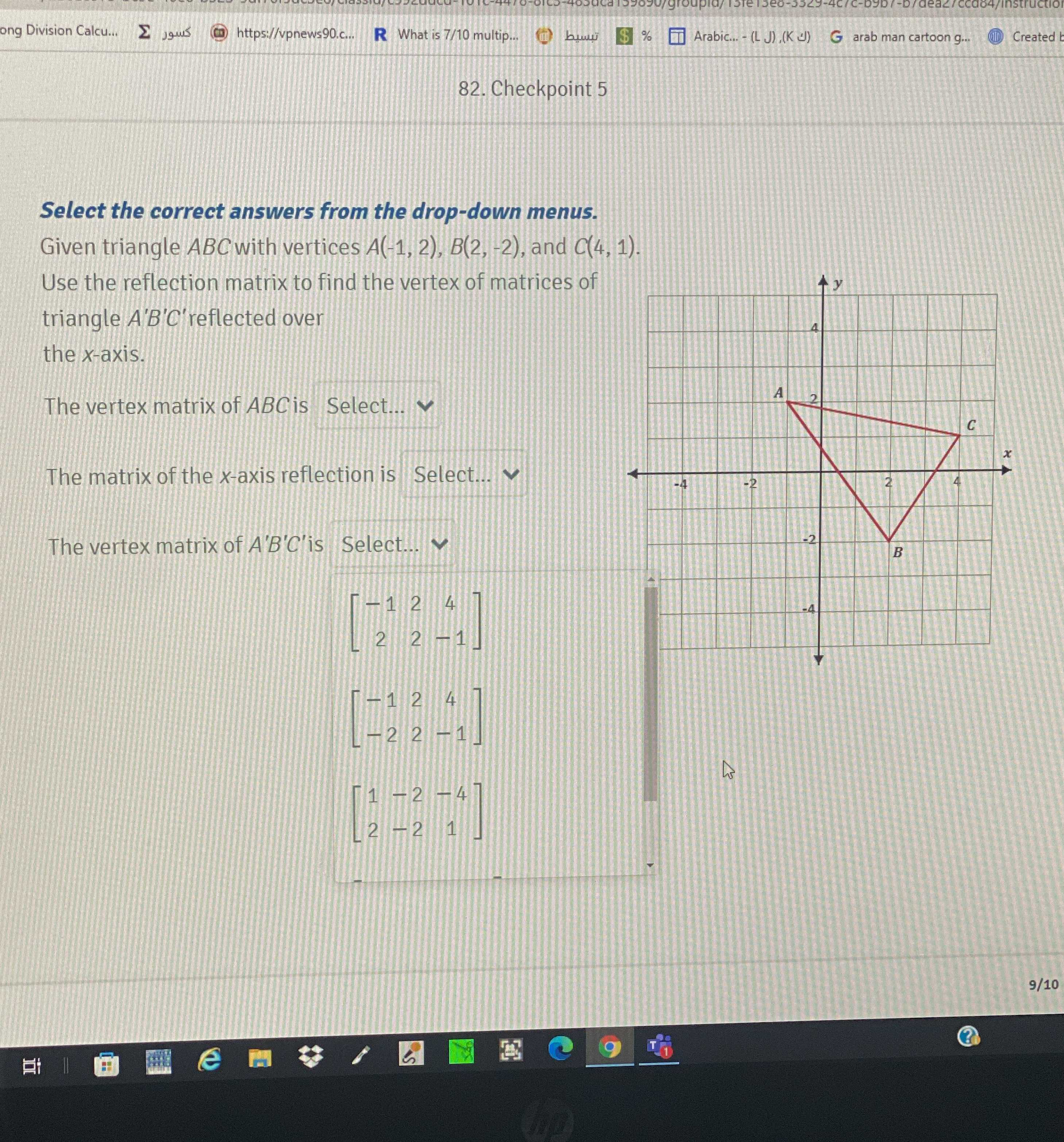### Still have math questions?

Algebra
QuestionGiven triangle $$A B C$$ with vertices $$A ( - 1,2 ) , B ( 2 , - 2 )$$ , and $$C ($$ Use the reflection matrix to find the vertex of matrices of triangle $$A ^ { \prime } B ^ { \prime } C ^ { \prime }$$ reflected over the $$x$$ -axis.

The vertex matrix of $$A B C$$ is Select...

The matrix of the $$x$$ -axis reflection is Select..

The vertex matrix of $$A ^ { \prime } B ^ { \prime } C ^ { \prime }$$ is Select...